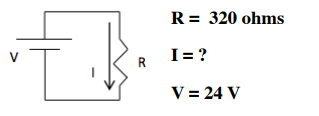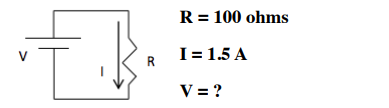Ch.13 - Fundamentals of ElectrochemistryWorksheetSee all chapters
 Ch.1 - Chemical Measurements 2hrs & 10mins 0% complete Worksheet Ch.2 - Tools of the Trade 1hr & 26mins 0% complete Worksheet Ch.3 - Experimental Error 1hr & 51mins 0% complete Worksheet Ch.4 + 5 - Statistics, Quality Assurance and Calibration Methods 2hrs & 1min 0% complete Worksheet Ch.6 - Chemical Equilibrium 3hrs & 43mins 0% complete Worksheet Ch.7 - Activity and the Systematic Treatment of Equilibrium 1hr & 1min 0% complete Worksheet Ch.8 - Monoprotic Acid-Base Equilibria 1hr & 52mins 0% complete Worksheet Ch.9 - Polyprotic Acid-Base Equilibria 2hrs & 16mins 0% complete Worksheet Ch.10 - Acid-Base Titrations 2hrs & 22mins 0% complete Worksheet Ch.11 - EDTA Titrations 1hr & 37mins 0% complete Worksheet Ch.12 - Advanced Topics in Equilibrium 1hr & 18mins 0% complete Worksheet Ch.13 - Fundamentals of Electrochemistry 2hrs & 27mins 0% complete Worksheet Ch.14 - Electrodes and Potentiometry 55mins 0% complete Worksheet Ch.15 - Redox Titrations 1hr & 14mins 0% complete Worksheet Ch.16 - Electroanalytical Techniques 1hr & 23mins 0% complete Worksheet Ch.17 - Fundamentals of Spectrophotometry 55mins 0% complete Worksheet BONUS: Chemical Kinetics Not available yet

# Basic Concepts

See all sections
Sections
Basic Concepts
Electrochemical Cells
Standard Potentials
Nernst Equation
Standard Cell Potential & the Equilibrium Constant
###### Basic Redox Concepts

Concept #1: OXIDATION-REDUCTION (REDOX) reactions deal with the transfer of electrons from one reactant to another.

Concept #2: The movement of electrons from one electrode to another helps in the generation of voltage.

###### Basic Redox Concepts Calculations

Example #1:

Example #2: Basic Redoxs Calculations 1

Practice: Solve for the missing variable in the following circuit.Practice: Solve for the missing variable in the following circuit.Example #3: Basic Redoxs Calculations 2

Example #4: Basic Redoxs Calculations 2

Practice: A metal forms the salt MCl 3. Electrolysis of the molten salt with a current of 0.700 A for 6.63 h produced 3.00 g of the metal. What is the molar mass of the metal?

Example #5: Basic Redoxs Calculations 3

Example #6: Basic Redoxs Calculations 3

Example #7: Basic Redoxs Calculations 3

###### Balancing Redox Reactions

Concept #3: Generally, you will need to balance a redox reaction in an acidic or basic solution.

Example #8: Balance the following redox reaction in acidic solution.

Cr2O72– (aq) + H2O2 (aq)  → Cr3+ (aq) + H2O (l) + O2 (g)

Practice: Balance the following redox reaction in basic solution.

Cr2O72– (aq) + SO32– (aq)  →  Cr3+ (aq)  +  SO42– (aq)